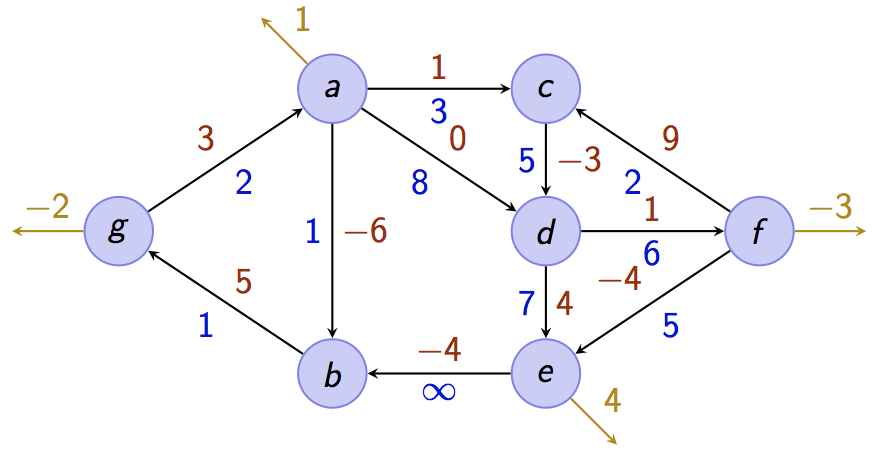max planck institut
informatik# Optimization (Summer Term 2015)

### Basic Information

Lecture time: Wed, 10-12 and Fri, 14-16
Lecture room: HS001, E1.3
Lecturers: Andreas Karrenbauer
Teaching Assistant Max John
Tutors Cornelius Brand, Davis Issac, Omar Darwish, Stephan Friedrichs
Good textbooks on the topic include:
Tutorials:
• Mondays, 8 AM with Stephan in MPII room 023
• Tuesdays, 4 PM with Cornelius in MPII room 023
• Thursdays, 2 PM with Omar in MPI-SWS room 630
• Fridays, 10 AM with Davis in MPII room 023
Prerequisites: Basics in linear algebra, discrete mathematics, calculus, algorithms, and complexity. At Saarland University these topics are covered in the bachelor courses Mathematik für Informatiker 1 & 2, Grundzüge der Theoretischen Informatik, and Grundzüge von Algorithmen und Datenstrukturen.
Exam Information: Your final grade will be the best of the final exam and the re-exam. You may bring one A4 cheat sheet (double-sided, in your own handwriting) to the exams.
Final Exam: July 29, 10-12 September 29, 13:30, Günter-Hotz-Hörsaal

### Announcements

• If you want to participate in the course, please register to our mailinglist.## Description

This course provides an introduction to fundamental concepts and algorithmic methods for solving linear and integer linear programs.

Linear optimization is a key subject in theoretical computer science. Moreover, it has many applications in practice. A lot of problems can be formulated as (integer) linear optimization problem. For example, combinatorial problems, such as shortest paths, maximum flows, maximum matchings in graphs, among others have a natural formulation as a linear (integer) optimization problem. In this course you will learn:

• how to optimize a linear function subject to linear constraints
• how to formulate combinatorial problems as (integer) linear optimization problems
• how to solve them

To this end, basic concepts from polyhedral theory will be introduced. The simplex algorithm and the ellipsoid method will be presented. The lecture concludes with exact and approximation algorithms for NP-hard optimization problems. There will be theoretical and practical exercises.### Policies

This is a 9-credit-point core lecture ("Stammvorlesung"). There will be two lectures and one exercise session per week. We will hand out exercises every week (usually worth 40 points) and each student should score at least 50% in the first half of the course (first 6 exercise sheets) and 50% in the second half in order to be allowed to take the exam.

You can also gain points by writing lecture notes of single lectures. In order to do so, you have to use our template. You gain 40γ points for the notes of one lecture, hereby γ denotes the quality factor in between 0 and 1 that will be determined by us. Please submit your notes by sending a zipped package that contains everything such that your tex code compiles and the pdf to Max not later than one week after the lecture that you took notes for. You can use the old notes from last year. If you need any tex source while writing your own notes, you can ask Max to send you the required parts.

## Lectures

The slides of all lectures will be condensed in a handout.

Date Topic Reference Homework Notes
Apr 22 Overview Exercise Sheet 1; Solution 1 Slides
Apr 24 Integer Programming Chapter 6 in [W] ; Chapter 11.8 in [BT] Slides; Scribe
Apr 29 Branch and Bound Chapter 7 in [W] ; Chapter 11.2 in [BT] Exercise Sheet 2; Solution 2 Slides; Scribe1; Scribe2
May 1 No lecture: Public holiday
May 6 Duality Chapter 4.7 in [BT] Exercise Sheet 3; Solution 3 Slides
May 8 Duality Slides; Scribe1; Scribe2
May 13 Fourier-Motzkin-Elimination, Farkas' Lemma Chapters 2.8 and 4.6 in [BT] Exercise Sheet 4; Solution 4 Slides; Scribe
May 15 Strong duality, Facets, Vertices Chapters 4.3 and 2.2 in [BT] Slides; Scribe1; Scribe2
May 20 Extreme points, vertices and basic feasible solutions Chapter 2.2 in [BT] Exercise Sheet 5; Solution 5 Slides; Scribe1; Scribe2
May 22 Optimality of extreme points, standard form Chapters 2.6 and 2.3 in [BT] Slides; Scribe1; Scribe2
May 27 Degeneracy, basic (feasible) directions, reduced costs Chapters 2.4 and 3.1 in [BT] Exercise Sheet 6; Solution 6 Slides; Scribe
May 29 Optimal bases, development of the Simplex method Chapters 3.1 and 3.2 in [BT] Slides
Jun 03 Simplex - The full tableau implementation Chapter 3.3 in [BT] Exercise Sheet 7; Solution 7 Slides; Scribe
Jun 05 Introduction to the Ellipsoid method - volume of polyhedra Chapter 8 in [BT] Slides; Scribe
Jun 10 The Ellipsoid method Chapter 8 in [BT] Exercise Sheet 8; Solution 8 Slides; Scribe
Jun 12 The Ellipsoid method Chapter 8 in [BT] Slides
Jun 17 Introduction to the interior point method Chapter 9 in [BT] Exercise Sheet 9; Solution 9 Slides; Scribe
Jun 19 The interior point method Chapter 9 in [BT] Slides; Scribe1; Scribe2
Jun 24 Integer optimization Chapter 3.2 in [W] Exercise Sheet 10; Solution 10 Slides; Scribe1; Scribe2
Jun 26 Generalization to polyhedra, unimodular matrices Chapter 3.2 in [W] Slides; Scribe1; Scribe2
Jul 01 Total unimodular matrices, network flows Chapters 7.3,7.4 in [BT] ; Chapters 3.2, 3.3 in [W] Exercise Sheet 11; Solution 11 Slides; Scribe1; Scribe2
Jul 03 Network flows Chapters 7.3,7.4 in [BT] ; Chapter 3.3 in [W] Slides; Scribe
Jul 08 Network flows Chapters 7.3,7.4 in [BT] ; Chapter 3.3 in [W] Exercise Sheet 12; Solution 12 Slides; Scribe
Jul 10 Recap, Interior point method for network flows Slides
Jul 15 Max-Flow Min-Cut, Bipartite Matching, Hall's theorem Chapter 8.2 in [MG] Exercise Sheet 13; Solution 13 Slides; Scribe
Jul 17 Matching polytopes, Approximation algorithms Chapter 25 in [S] Slides; Scribe
Jul 22 Approximation algorithms Test exam Slides
Jul 24 Max-Cut and SDP relaxations Slides
Jul 29 Final exam E2.5, HS 1
Jul 31 Possibly exam review
Sep 29 Re-exam (start time: 1:30pm) Günther-Hotz-Hörsaal

More Courses of the Algorithms and Complexity Group